Summary: in this tutorial, you will learn about C linked list data structure and how to implement the most commonly used linked list operations.

Introduction to linked list data structure

A linked list is a data structure that consists of sequence of nodes. Each node is composed of two fields: data field and reference field which is a pointer that points to the next node in the sequence.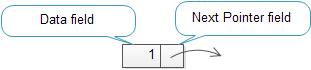Each node in the list is also called an element. The reference field that contains a pointer which points to the next node is called next pointer or next link.

A head pointer is used to track the first element in the linked list, therefore, it always points to the first element.

The following picture illustrates a linked list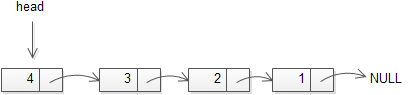The linked list data structure is designed to be efficient for insertion or removal of elements from any position in the list. However other operations such as getting the last element or finding an element that stores specific data requires scanning most or all the elements in the list.

A linked list is also used to implement other data structure such as stack and queue.

C Linked List implementation

We can model a node of the linked list using a structure as follows:

The node structure has two members:

• data stores the information
• next pointer holds the address of the next node.

Add a node at the beginning of the linked list

First, we declare a head pointer that always points to the first node of the list.

To add a node at the beginning of the list:

First, we need to create a new node. We will need to create a new node each time we want to insert a new node into the list so we can develop a function that creates a new node and return it.

Second, we need to point the next pointer of the new node to the head pointer and point the head pointer to the new node. It works for both empty and non-empty linked list.

The following picture illustrates how to insert a node when the list is empty:

The following picture illustrates how to insert a node at the beginning of a non-empty linked list:

Traverse the linked list

Sometimes we may want to traverse the linked list to get the data stored in each node for further manipulation e.g., display the node information. To traverse the linked list, we start from the first node, and move to the next node until we reach a NULL pointer.

To make the traverse() function more general, we can add a function pointer that points to a function for linked list node manipulation as a parameter of the traverse() function.

We define a call back function for manipulating a node of the linked list:

The following is the traverse() function:

Count the elements of the linked list

We can use the same traversing technique to count the number of elements in a linked list. See the following count() function:

Add a new node at the end of the linked list

To add a new node at the end of the linked list:

First, we need to find the last node of the list. Starting the first node which is indicated by the head pointer, we traverse the list until the next pointer reaches NULL.

Second, we create a new node with the next pointer points to NULL , and the next pointer of the cursor points to the new node: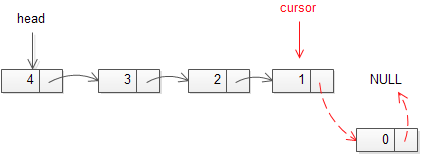Add a new node at the end of the linked list

The following is the append() function that adds a new node at the end of the linked list.

Insert a new node after a particular node

To insert a new node after a particular node, we need to:

First, verify if the node exists in the list, we call this node is prev node

If it exists, we point the next pointer of the new node to the next node that the next pointer of the prev node points to, and point the next pointer of the prev node to the new node.

The following picture illustrates how to insert a new node after a particular node in the linked list: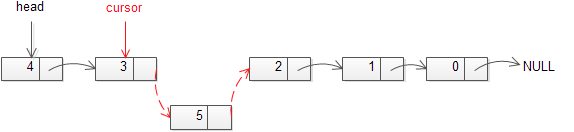Insert a new after a particular node in the linked list

The insert_after() function is as follows:

Insert a new node before a particular node

To insert a new node before a particular node named nxt we need to:

• First, if the nxt node is the first node, we can call the prepend() function to add a new node at the beginning of the list, otherwise, we find the previous node of the nxt node, suppose it is the cursor node.
• Second, point the next pointer of the new node to the node that the next pointer of the cursor points to and point the next pointer of the cursor to the new node.

The following picture illustrates how to insert a node before a particular node: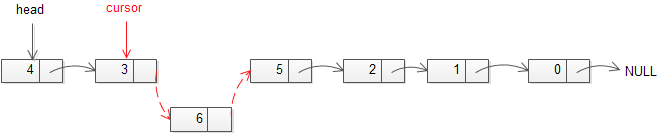Insert a node before a particular node

The following is the insert_before() function that inserts a new node before a particular node in the linked list:

Search for a node

To search for a node that stores a given data, we scan the whole list and return the first node that stores the searched data. The following illustrates the search function:

The search() function returns NULL if no node stores the input data.

Sort a linked list using insertion sort

We can sort a linked list using the insertion sort algorithm. The following is the insertion_sort() function that sorts a linked list:

To reverse a linked list, you change the next pointer of each node from the next node to the previous node. The following reverse() function reverses a linked list.

Delete a node from the front of the linked list

To delete a node from the front of the linked list is relatively simple. We point the head to the next node and remove the node that the head pointed to.

Notice that if the list has only one node, we should set the head pointer to NULL.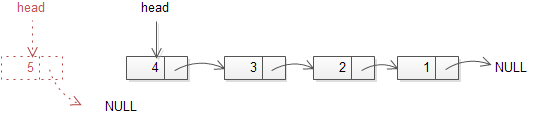Remove from the front of the list

The following is the remove_front() function:

Delete a node from the back of the linked list

To remove a node from the back of the linked list, we need to:

• Use two pointers: cursor and back to track the node.
• Start from the first node until the cursor pointer reaches the last node and the back pointer reaches the node before the last node.
• Set the next pointer of the back to NULL and delete the node that the cursor points to.
• If the node has only 1 element, set the head pointer to NULL before removing the node.

See the following picture: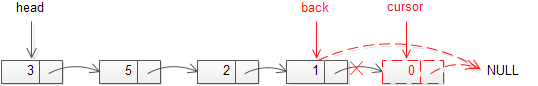Delete a node from the back of the linked list

The following is the function to remove a node from the back of the linked list:

Delete a node in the middle of the linked list

To delete a node in the middle of the linked list:
If the node is the first node, call remove_front() function to remove it.
If the node is the last node, call remove_back() function to remove it.
If the node is in the middle of the list:

• Traverse from the first node, use the cursor pointer to point to the node before the node that needs to be removed e.g., in the picture, the node that needs to be removed is 2 and the cursor pointer points to node 5.
• Use a tmp pointer to point to the node that needs to be removed.
• Set the next pointer of the cursor point to the node that the next pointer of the tmp points to.
• Remove the node that the tmp pointer points to.

The following picture illustrates how to delete a node in the middle of the linked list: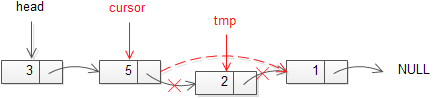Remove the middle node of a linked list

The following is the function that can be used to remove any node in the linked list:

Delete the whole linked list

It is important to remove all nodes of the linked list when you no longer use it. The following dispose() function releases memory allocated for all the nodes in a linked list:

C Linked List Program

We can write a program to test the linked list functions that we’ve developed in the above section.

The following is the output when we ran the C linked list program above: